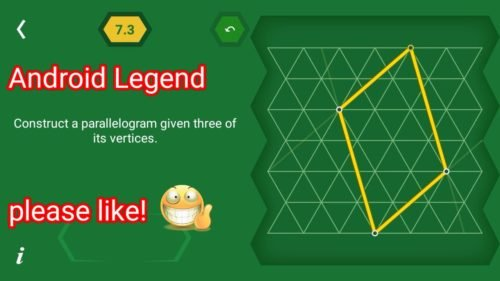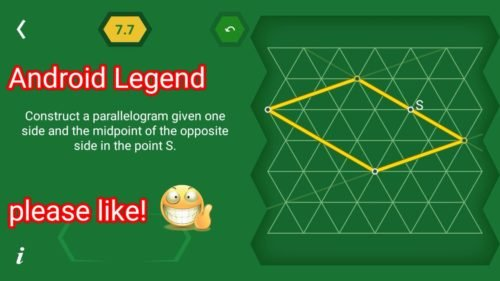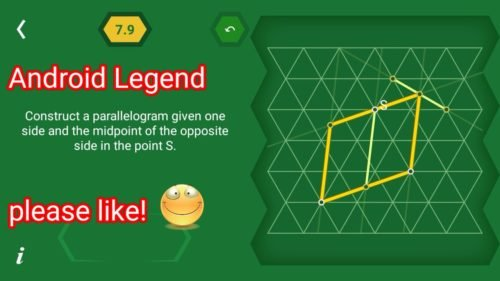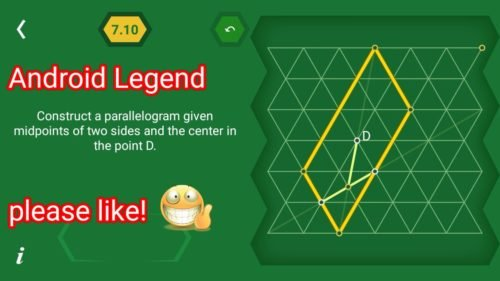# Pythagorea 60 degree Level 7.1 7.2 7.3 7.4 7.5 7.6 7.7 7.8 7.9 7.10 Solution/AnswersSolutions, Hints, and Answers to pythagorea 60° are available in this post, Scroll down to find solutions to all the levels.

Pythagorea sixty degrees is android/iOS app developed by Horis International Limited. This game is mostly focused on geometric puzzles and construction. The workspace is divided into triangular grids lines aligned 60° to each other, that’s why this game names as pythagorea 60°. You should know all the basic Math operations. All lines and shapes are drawn on a grid whose cells are equilateral triangles. Most of the game levels can be answered using natural intuition and bye some basic laws of geometry.

You have to connect points on the grid using straight lines to construct an element, you can even use intersection points to draw. some levels are very easy some are of medium difficulty and some are very hard to solve, that’s why I am providing solutions to all the problems.

Download link for Android devices: https://play.google.com/store/apps/details?id=com.hil_hk.pythagorea60

Download link for iOS devices: https://itunes.apple.com/us/app/pythagorea-60/

If you are here for levels other than ‘Parallelogram’ Go to directory of all other levels at : http://www.puzzlegamemaster.com/pythagorea-60-complete/

• Pythagorea 60° Level 7.1: Connect the four given points to get a parallelogram.• Pythagorea 60° Level 7.2: Construct a parallelogram given three of its vertices.• Pythagorea 60° Level 7.3: Construct a parallelogram given three of its vertices.• Pythagorea 60° Level 7.4: Construct a parallelogram given the midpoints of its sides• Pythagorea 60° Level 7.5: Construct a parallelogram given one side and the Center in the point D.• Pythagorea 60° Level 7.6: Construct a parallelogram given one side and the Center in the point D.• Pythagorea 60° Level 7.7: Construct a parallelogram given one side and the midpoint of the opposite side in the point S.• Pythagorea 60° Level 7.8: Construct a parallelogram given one side and the midpoint of the opposite side in the point S.• Pythagorea 60° Level 7.9: Construct a parallelogram given one side and the midpoint of the opposite side in the point S.• Pythagorea 60° Level 7.10: Construct a parallelogram given midpoints of two sides and the center in the point D.Feel free to comment below if you have any doubts regarding the solutions. I will try to help you guys. All other levels are posted on this blog please visit them too.